Ex 15.1

Chapter 15 Class 10 Probability
Serial order wise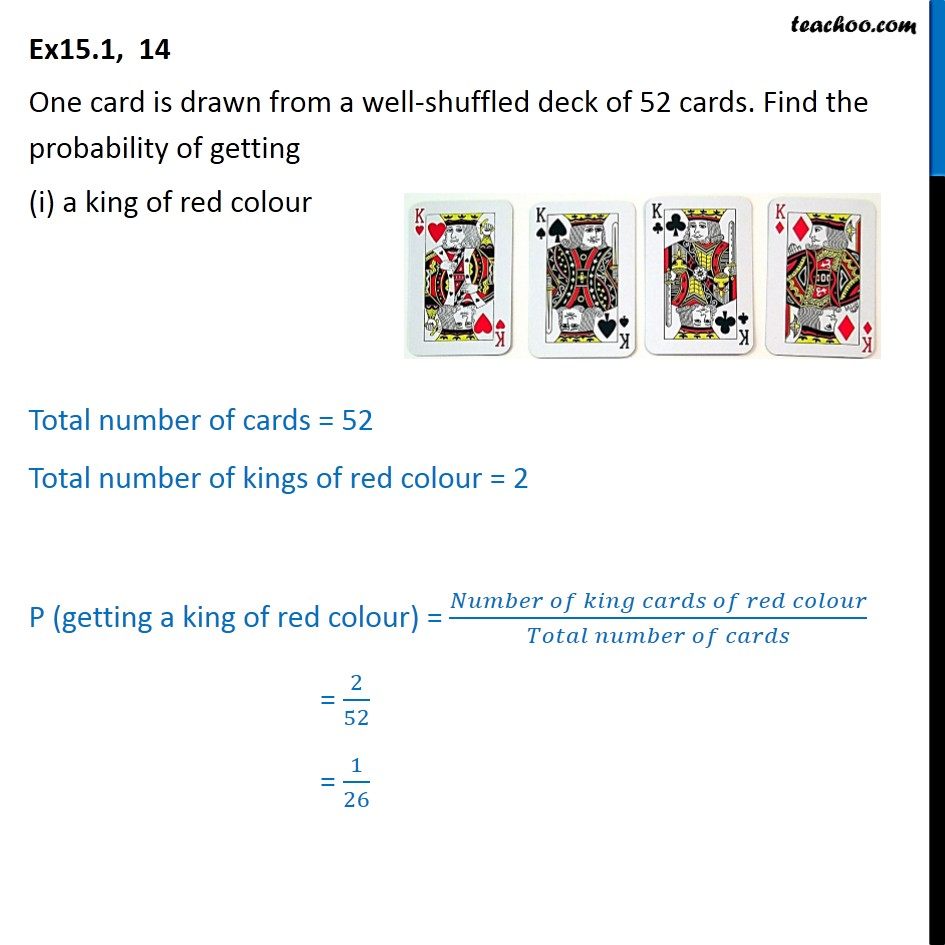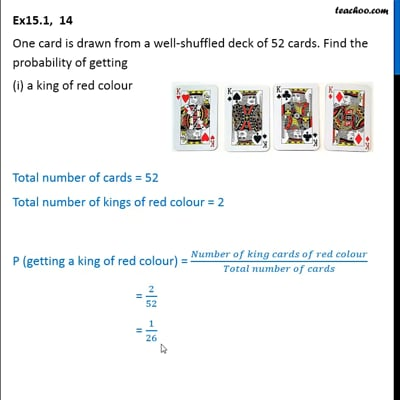This video is only available for Teachoo black users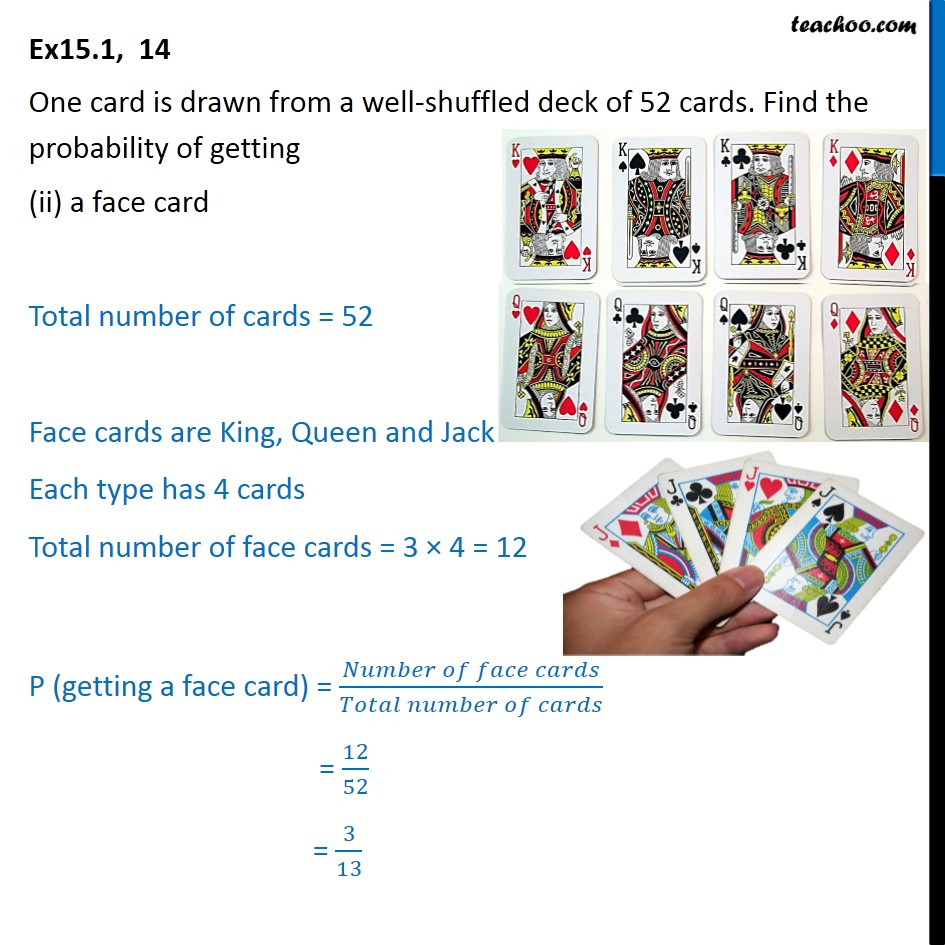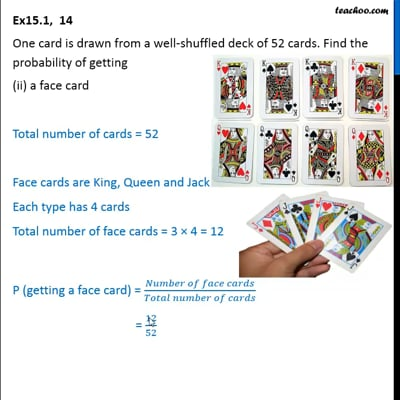This video is only available for Teachoo black users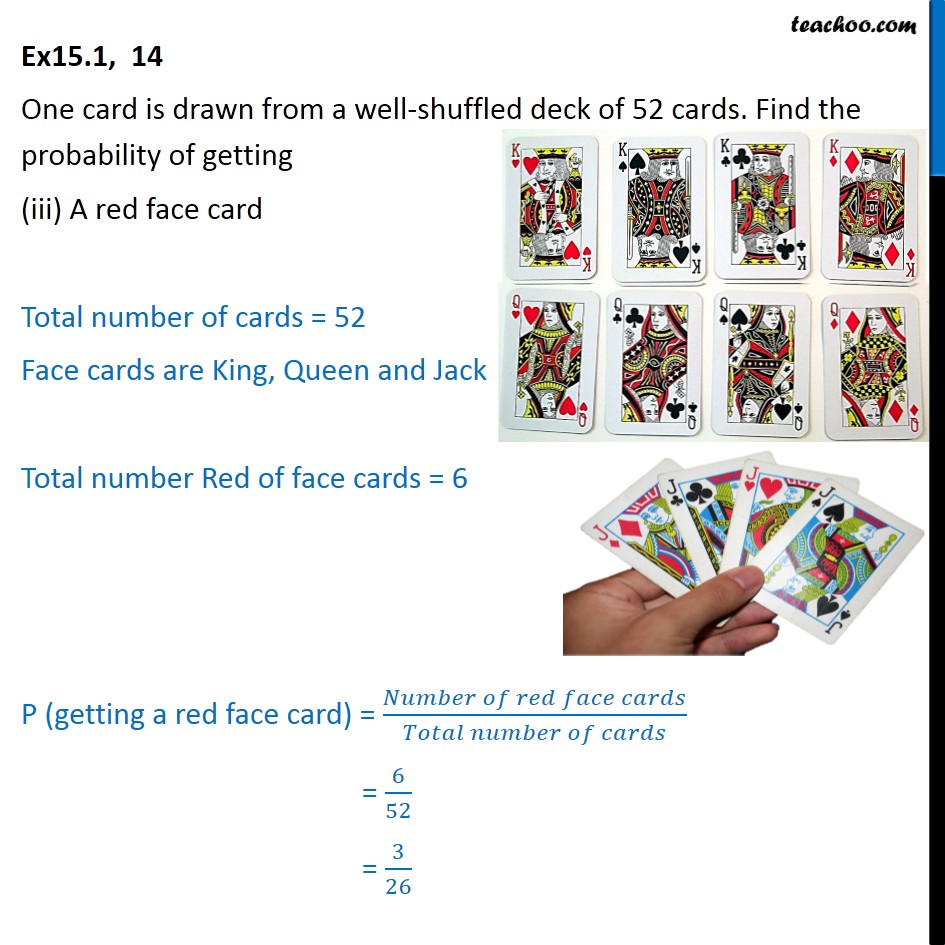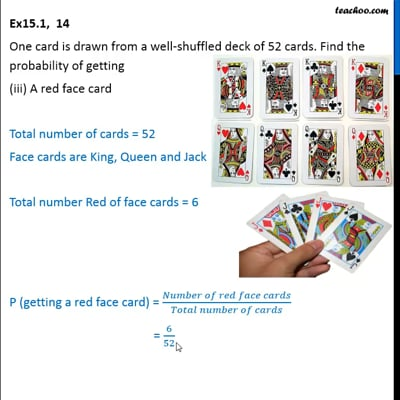This video is only available for Teachoo black users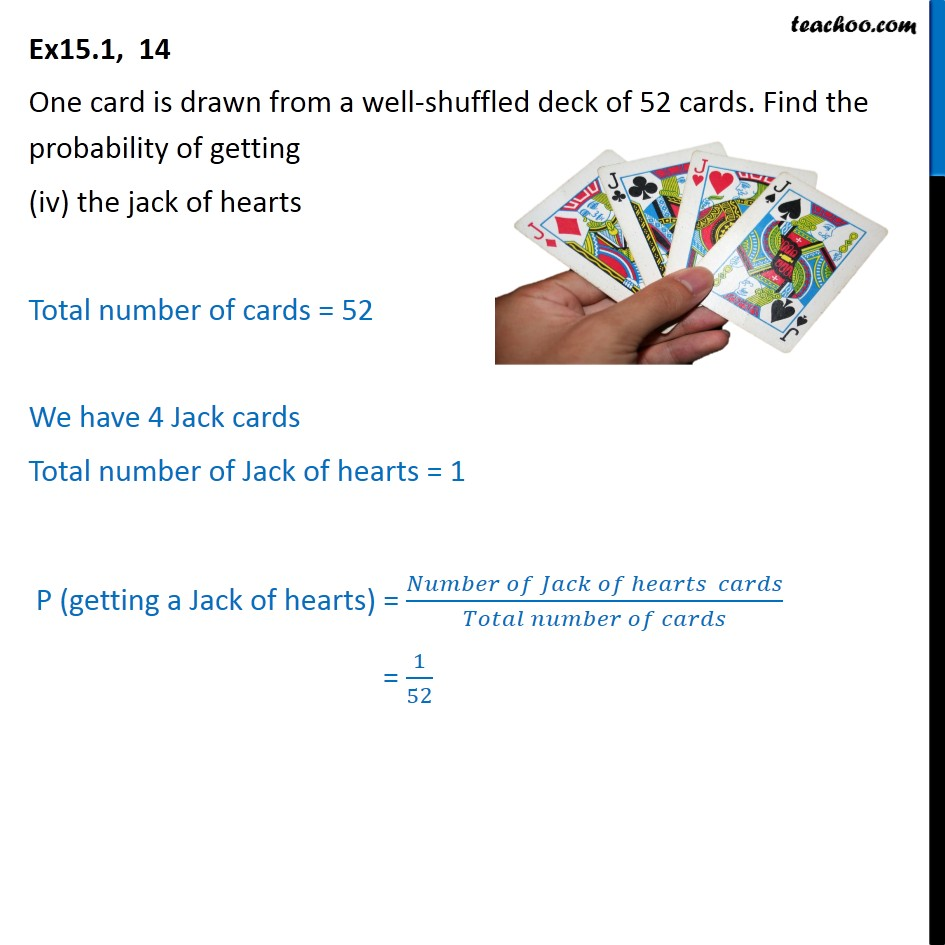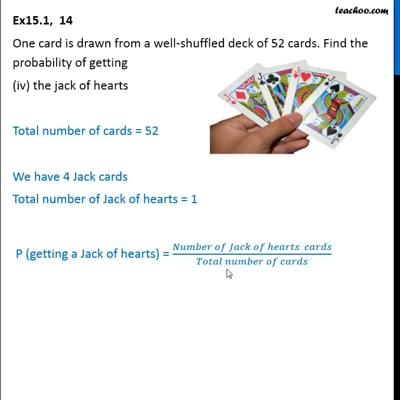This video is only available for Teachoo black users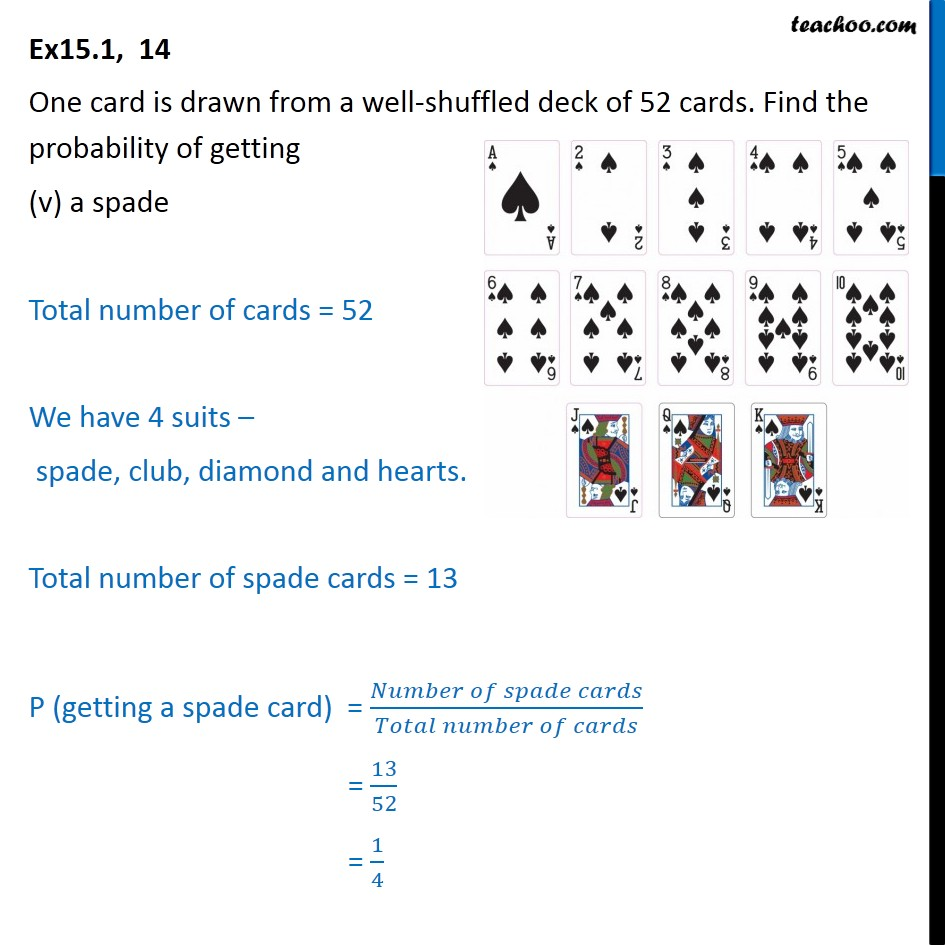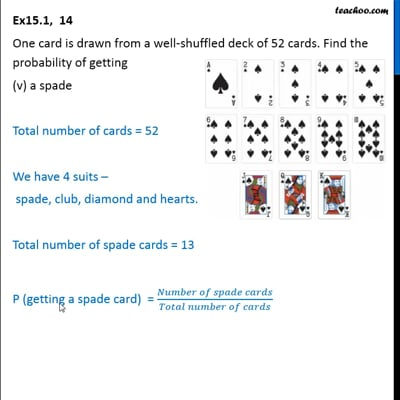This video is only available for Teachoo black users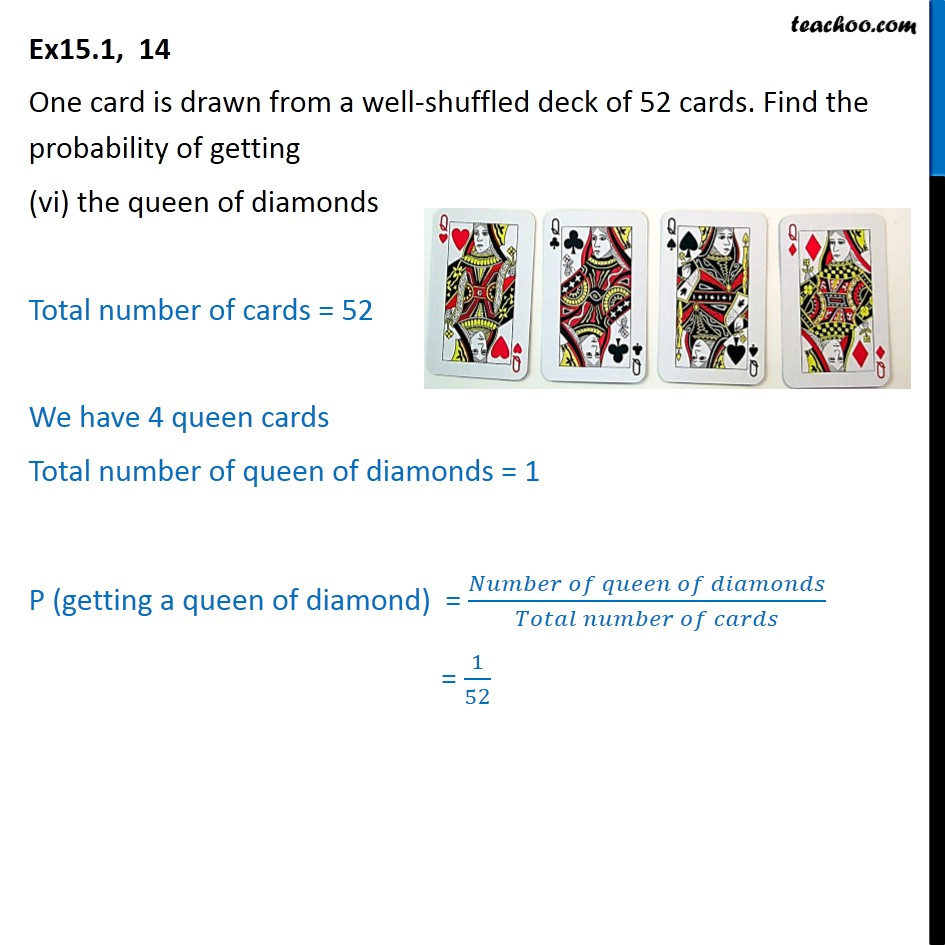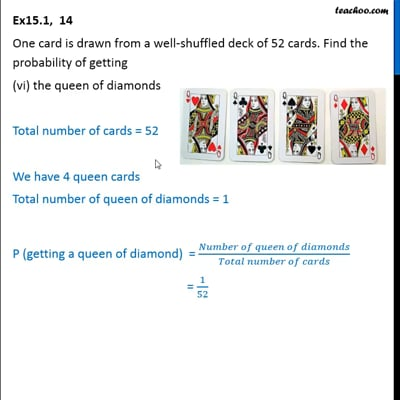This video is only available for Teachoo black users

Solve all your doubts with Teachoo Black (new monthly pack available now!)

### Transcript

Ex15.1, 14 One card is drawn from a well-shuffled deck of 52 cards. Find the probability of getting (i) a king of red colour Total number of cards = 52 Total number of kings of red colour = 2 P (getting a king of red colour) = (𝑁𝑢𝑚𝑏𝑒𝑟 𝑜𝑓 𝑘𝑖𝑛𝑔 𝑐𝑎𝑟𝑑𝑠 𝑜𝑓 𝑟𝑒𝑑 𝑐𝑜𝑙𝑜𝑢𝑟)/(𝑇𝑜𝑡𝑎𝑙 𝑛𝑢𝑚𝑏𝑒𝑟 𝑜𝑓 𝑐𝑎𝑟𝑑𝑠) = 2/52 = 1/26 Ex15.1, 14 One card is drawn from a well-shuffled deck of 52 cards. Find the probability of getting (ii) a face card Total number of cards = 52 Face cards are King, Queen and Jack Each type has 4 cards Total number of face cards = 3 × 4 = 12 P (getting a face card) = (𝑁𝑢𝑚𝑏𝑒𝑟 𝑜𝑓 𝑓𝑎𝑐𝑒 𝑐𝑎𝑟𝑑𝑠)/(𝑇𝑜𝑡𝑎𝑙 𝑛𝑢𝑚𝑏𝑒𝑟 𝑜𝑓 𝑐𝑎𝑟𝑑𝑠) = 12/52 = 3/13 Ex15.1, 14 One card is drawn from a well-shuffled deck of 52 cards. Find the probability of getting (iii) A red face card Total number of cards = 52 Face cards are King, Queen and Jack Total number Red of face cards = 6 P (getting a red face card) = (𝑁𝑢𝑚𝑏𝑒𝑟 𝑜𝑓 𝑟𝑒𝑑 𝑓𝑎𝑐𝑒 𝑐𝑎𝑟𝑑𝑠)/(𝑇𝑜𝑡𝑎𝑙 𝑛𝑢𝑚𝑏𝑒𝑟 𝑜𝑓 𝑐𝑎𝑟𝑑𝑠) = 6/52 = 3/26 Ex15.1, 14 One card is drawn from a well-shuffled deck of 52 cards. Find the probability of getting (iv) the jack of hearts Total number of cards = 52 We have 4 Jack cards Total number of Jack of hearts = 1 P (getting a Jack of hearts) = (𝑁𝑢𝑚𝑏𝑒𝑟 𝑜𝑓 𝐽𝑎𝑐𝑘 𝑜𝑓 ℎ𝑒𝑎𝑟𝑡𝑠 𝑐𝑎𝑟𝑑𝑠)/(𝑇𝑜𝑡𝑎𝑙 𝑛𝑢𝑚𝑏𝑒𝑟 𝑜𝑓 𝑐𝑎𝑟𝑑𝑠) = 1/52 Ex15.1, 14 One card is drawn from a well-shuffled deck of 52 cards. Find the probability of getting (v) a spade Total number of cards = 52 We have 4 suits – spade, club, diamond and hearts. Total number of spade cards = 13 P (getting a spade card) = (𝑁𝑢𝑚𝑏𝑒𝑟 𝑜𝑓 𝑠𝑝𝑎𝑑𝑒 𝑐𝑎𝑟𝑑𝑠)/(𝑇𝑜𝑡𝑎𝑙 𝑛𝑢𝑚𝑏𝑒𝑟 𝑜𝑓 𝑐𝑎𝑟𝑑𝑠) = 13/52 = 1/4 Ex15.1, 14 One card is drawn from a well-shuffled deck of 52 cards. Find the probability of getting (vi) the queen of diamonds Total number of cards = 52 We have 4 queen cards Total number of queen of diamonds = 1 P (getting a queen of diamond) = (𝑁𝑢𝑚𝑏𝑒𝑟 𝑜𝑓 𝑞𝑢𝑒𝑒𝑛 𝑜𝑓 𝑑𝑖𝑎𝑚𝑜𝑛𝑑𝑠)/(𝑇𝑜𝑡𝑎𝑙 𝑛𝑢𝑚𝑏𝑒𝑟 𝑜𝑓 𝑐𝑎𝑟𝑑𝑠) = 1/52